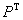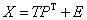Home News About Me Ph. D. Thesis Abstract Table of Contents 1. Introduction 2. Theory  Fundamentals of the Multivariate Data Analysis 2.1. Overview of the Multivariate Quantitative Data Analysis 2.2. Experimental Design 2.3. Data Preprocessing 2.4. Data Splitting and Validation 2.5. Calibration of Linear Relationships 2.6. Calibration of Nonlinear Relationships 2.7. Neural Networks  Universal Calibration Tools 2.8. Too Much Information Deteriorates Calibration 2.8.1. Overfitting, Underfitting and Model Complexity 2.8.2. Neural Networks and the Complexity Problem 2.8.3. Brute Force Variable Selection 2.8.4. Variable Selection by Stepwise Algorithms 2.8.5. Variable Selection by Genetic Algorithms 2.8.6. Variable Selection by Simulated Annealing 2.8.7. Variable Compression by Principal Component Analysis 2.8.8. Topology Optimization by Pruning Algorithms 2.8.9. Topology Optimization by Genetic Algorithms 2.8.10. Topology Optimization by Growing Neural Network Algorithms 2.9. Measures of Error and Validation 3. Theory  Quantification of the Refrigerants R22 and R134a: Part I 4. Experiments, Setups and Data Sets 5. Results  Kinetic Measurements 6. Results  Multivariate Calibrations 7. Results  Genetic Algorithm Framework 8. Results  Growing Neural Network Framework 9. Results  All Data Sets 10. Results  Various Aspects of the Frameworks and Measurements 11. Summary and Outlook 12. References 13. Acknowledgements Publications Research Tutorials Downloads and Links Contact Search Site Map Print this Page### 2.8.7.   Variable Compression by Principal Component Analysis

The Principal Component Analysis (PCA), which originates from psychometrics, can be used as preprocessing tool for neural networks. Thereby the PCA compresses the independent variables into fewer principal components, which are then used as new input variables for the neural networks. The PCA finds the direction in space along which the variance of the data is the largest. This direction is called the first principal component. The second principal component is the direction in space orthogonal to the first principal component, which describes maximum variance not covered by the first principal component, and so on. The data matrix is decomposed by the PCA into a product of a loading matrixand of a sore matrix T and a matrix containing the residuals E:(19)

Similar to the PLS only the first few principal components are used with similar methods to determine the optimal number (see section 2.5).

Yet, the variable compression by principal components is affected by some (at least theoretical) drawbacks. Using only few principal components does not ensure that the information preserved in these components is useful for the calibration of the relationship of interest. For example, if noise dominates the variations of the input variables, the variations caused by the sensor responses due to the analytes might not be included as the corresponding principal components with small singular values are discarded . Additionally, nonlinear relationships are often spread over many principal components, which might not be included in the model (see also discussions in the sections 6.1 and 9.2.5). As in contrast to the PLS the principal components are determined only on the basis of the variances of the independent variables and not on the basis of an optimal regression, no synergetic effects of the combi­nation of the PCA and the neural networks can be expected.

 Page 54 © Frank Dieterle, 03.03.2019